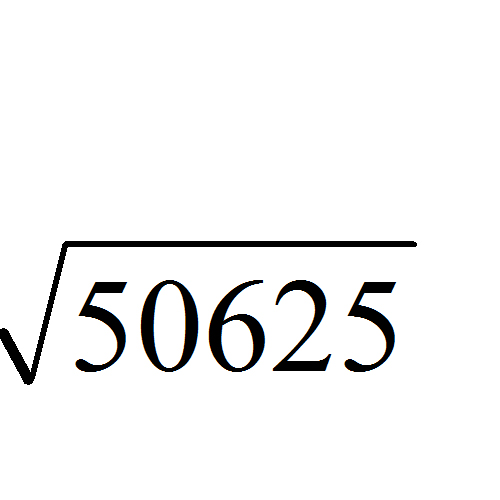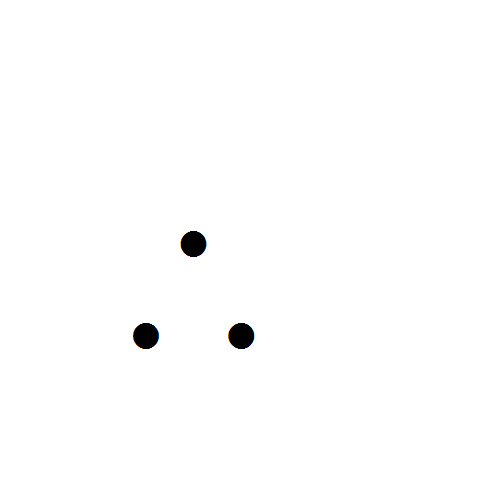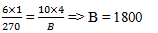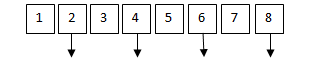# Sample Aptitude Questions of NIIT-Technologies

1. A number X is 150 more than a second number, Y. If the sum of X and Y is 5 times Y, what is the value of Y ?
1. 50
2. 40
3. 80
4. 60
5. 70

X = 150 + Y
X + Y = 5Y
150 + 2Y = 5Y
150 = 3Y => Y = 502. A square field has an area of 50625 m2. Find the cost of fencing around it at Rs. 15 per metre, (in Rs.)
1. 12,500
2. 6750
3. 17,500
4. 13500
5. 16250

Area of square field = 50625 => side of the field == 225cost of fencing @ Rs. 15/ meter  = 4 × 225 × 15 = Rs. 13500
1. The average weight of 8 person's increases by 2.5 kg when a new person comes in place of one of them weighing 65 kg. What will be the weight of the new person ?
1. 76
2. 76.5
3. 85
4. 80
5.  90

Increased wt. of 8 persons = 8 × 2.5 = 20 kg
=>wt. of new person = 65 + 20 = 85 kg
2. 50% of a number is 18 less than two-third of that number. Find the number ?
1. 123
2. 115
3. 119
4. 108
5. 101

Let the no. be x
Given : 50x/100  = 2x/3 – 18
½ x – 2/3 x = - 18
=> x = 108
3. Running at the same constant rate, 6 identical machines can produce a total of 270 bottles per minute. At this rate, how many bottles could 10 such machines produce in 4 minutes?
1. 648
2.  1800
3. 2700
4. 2000
5. 3080

Let the no. of bottles be ‘B’. Using chain rule:4. 652.84 + 482.26 + ? = 1200
1. 62.16
2.  54.18
3. 56.1
4.  64.9
5.  66.1

652.84 + 482.26 + x  = 1200,=> 1135.1 + x = 1200 => x = 64.9
5. 60% of 250 -? = 75
1. 25
2.  45
3. 60
4. 75
5.  100

=>60% of 250 - x = 75
= 150 – x = 75 => x = 75
6. 681 + ? * 40 = 1161
1. 14
2. 12
3. 24
4. 16
5.  8

681 + x × 40 = 1161
= 681 + 40x = 1161 => 40x = 1161 – 681 = 480
=> x = 480/40 = 127. Different words are formed with the help of the letters of word RELATION. Find the number of ways in which vowels always occupy even places.
1. 292
2.  654
3. 356
4. 576
5. 8!